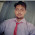## Tuesday, January 17, 2012

### Simple Ratio problem that often confuses

Sum of three nos is 98. The ratio between 1 and 2 is 2:3. The ratio between 2 and 3 is 5:8 .
Find the second no?

Let x,y,z be numbers.
x/y=2/3 , So, 3x=2y. And y/z=5/8 , So 8y=5z
Sum is 98, So 2y/3+y+8y/5=98. Therefore y=30
Numbers are 20,30,48

1.HI
Very best articles, I happy to read it, Beautiful nice and useful!
Thank you very much

1.Be blessed :)

2.Hi there,
Simple Ratio problem that often confuses
Fantastic blog post, I wish to apprentice at the same time how you amend your website.

1.Pls talk in detail...

3.one of the Ratio based que which come in every exams, good for practice